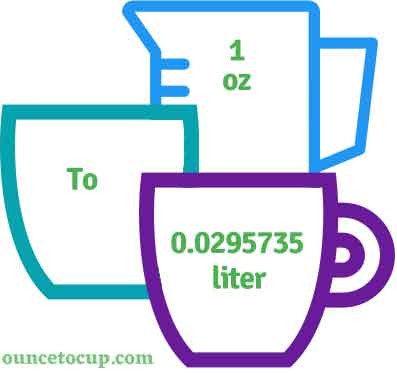# Ounce to Liter (oz to l conversion)

Are you cooking your favorite dish? The detailed chart in the recipe includes the calculation of 1 ounces to l conversion? Do not worry; check this conversion tool to find how many 1 ounces equal to liter in a minute. This 1 oz to liter converter gives an exact measurement for any recipe you prepare.

Ounce Value:

oz

Liter Value:

l

1 Ounce = 0.0295735 Liter
(1 oz = 0.0295735 l)

Try our auto 1 ounce to liter calculator (Without Convert Button), Just change the first field value and you got final value.## How many l is a oz?

We know that the volume value of 1 oz is equal to 0.0295735 l. If you want to convert 1 fluid oz to an equal number of l, just multiply the volume value by 0.0295735. Hence, 1 Ounce is equal to 0.0295735 l.

The Answer is: 1 US Fluid Ounces = 0.0295735 US l

1 oz = 0.0295735 l

Many of them try to search or find an answer for what is 1 ounces in l? So, we’ll start with 1 fl to oz l conversion to know how big is 1 oz.

## How To Calculate 1 fluid oz to liter?

To calculate 1 fluid ounces to an equal number of liter, simply follow the steps below.

Fluid Ounces to Liters formula is:

Liter = Fluid Ounce * 0.0295735

Assume that we are finding out how many l were found in 1 fl oz of water, multiply by 0.0295735 to get the result.

Applying to Formula: l = 1 oz * 0.0295735 = 0.0295735 l.

## How To Convert oz to liter?

• To convert fluid ounces to liter,
• Simply multiply the fluid ounce value by 0.0295735.
• Applying to the formula, l = 1 ounces * 0.0295735 [1x0.0295735].
• Hence, 1 ounces is equal to 0.0295735 l.

## Some quick table references for ounce to liter conversions:

Ounce [oz]Liter [l]
1 oz0.0295735 l
2 oz0.0591 l
3 oz0.0887 l
4 oz0.1183 l
5 oz0.1479 l
6 oz0.1774 l
7 oz0.207 l
8 oz0.2366 l
9 oz0.2662 l
10 oz0.2957 l
11 oz0.3253 l
12 oz0.3549 l
13 oz0.3845 l
14 oz0.414 l
15 oz0.4436 l

## Reverse Calculation: How many ounces are in a l?

• To convert 1 l to oz,
• Simply multiply the 1 liter by 33.814.
• Then, applying the formula, ounce = 1 l * 33.814 [1x33.814 = 133.814].
• Hence, 1 liter is equal to 33.814 oz.

### Related Converter:

Formula: Ounce to liter

l = ounce * 0.0295735

Applying to Formula,

l = 1*0.0295735 = 0.0295735

1 oz = 0.0295735 l